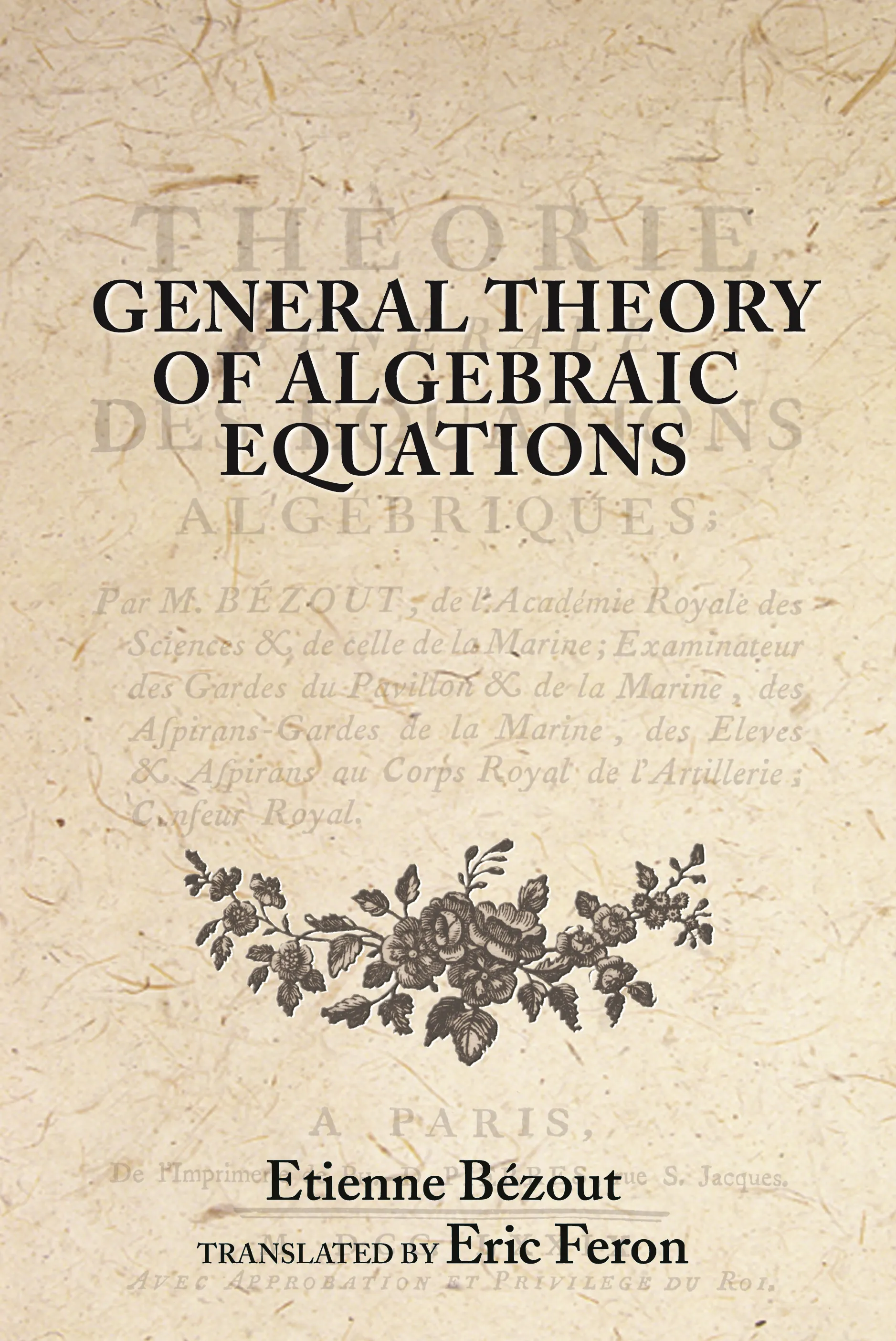#### General Theory of Algebraic Equations

Etienne Bézout

Audiobooks and ebooks purchased from this site must be accessed on the Princeton University Press app.

# General Theory of Algebraic Equations

Translated by
• Eric Feron## Hardcover

Price:
\$95.00/£80.00
ISBN:
Published:
Apr 2, 2006
2006
Pages:
368
Size:
6 x 9.25 in.

This book provides the first English translation of Bezout’s masterpiece, the General Theory of Algebraic Equations. It follows, by almost two hundred years, the English translation of his famous mathematics textbooks. Here, Bézout presents his approach to solving systems of polynomial equations in several variables and in great detail. He introduces the revolutionary notion of the “polynomial multiplier,” which greatly simplifies the problem of variable elimination by reducing it to a system of linear equations. The major result presented in this work, now known as “Bézout’s theorem,” is stated as follows: “The degree of the final equation resulting from an arbitrary number of complete equations containing the same number of unknowns and with arbitrary degrees is equal to the product of the exponents of the degrees of these equations.”

The book offers large numbers of results and insights about conditions for polynomials to share a common factor, or to share a common root. It also provides a state-of-the-art analysis of the theories of integration and differentiation of functions in the late eighteenth century, as well as one of the first uses of determinants to solve systems of linear equations. Polynomial multiplier methods have become, today, one of the most promising approaches to solving complex systems of polynomial equations or inequalities, and this translation offers a valuable historic perspective on this active research field.